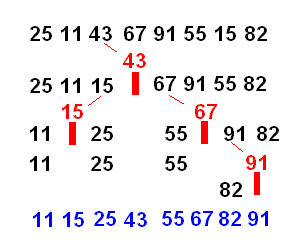# Quicksort

Also found in: Wikipedia.

## Quicksort

A sorting algorithm with O(n log n) average time complexity.

One element, x of the list to be sorted is chosen and the other elements are split into those elements less than x and those greater than or equal to x. These two lists are then sorted recursively using the same algorithm until there is only one element in each list, at which point the sublists are recursively recombined in order yielding the sorted list.

This can be written in Haskell:

qsort :: Ord a => [a] -> [a] qsort [] = [] qsort (x:xs) = qsort [ u | u<-xs, u<x ] ++ [ x ] ++ qsort [ u | u<-xs, u>=x ]

[Mark Jones, Gofer prelude.]

## quick sort

A sorting technique that sequences a list by continuously dividing the list into two parts and moving the lower items to one side and the higher items to the other. It starts by picking one item in the entire list to serve as a pivot point. The pivot could be the first item or a randomly chosen one. All items that compare lower than the pivot are moved to the left of the pivot; all equal or higher items are moved to the right. It then picks a pivot for the left side and moves those items to left and right of the pivot and continues the pivot picking and dividing until there is only one item left in the group. It then proceeds to the right side and performs the same operation again. See sort algorithm.

Quick Sorting a ListThis quick sort uses randomly chosen pivots (in red) to keep dividing the list into two until there is only one item on each side of the pivot left.
Copyright © 1981-2019 by The Computer Language Company Inc. All Rights reserved. THIS DEFINITION IS FOR PERSONAL USE ONLY. All other reproduction is strictly prohibited without permission from the publisher.
Mentioned in ?
References in periodicals archive ?
a Quicksort algorithm in which the partitioning phase uses one pivot to split a given array into two subarrays ).
Then the quicksort approach is adopted to select the eligible terminals and the complexity is O([K.sup.2][log.sub.2][K.sup.2]).
(1) Sort the objective vectors on every objective by using Quicksort .
Obviously, traditional sorting algorithms (e.g., merge sort, quicksort, and heap sort) cannot solve the problem of sorting encrypted data items, but by introducing the proposed secure comparison model, the encrypted data will be sorted by using the corresponding comparable codes.
Parallelizing Quicksort Algorithm using JOMP and its performance analysis with OpenMP.
Tsigas, "GPU-quicksort: A practical quicksort algorithm for graphics processors", Journal of Experimental Algorithmics, vol.
Note that the Quicksort method is utilized in MFO and the sort's computational complexity is O(n log n) and O([n.sup.2]) in the best and worst case, respectively (where n is the number of moths).
It consists in evaluating the run-times of three well-known sorting algorithms: QuickSort, BubbleSort and InsertSort.
After computing the [n.sub.[phi]] value of the list of recommended articles, we implement a quicksort algorithm on these articles according to the [n.sub.[phi]].
When there are no overlapped wavelength shifts, the efficiency of traditional algorithm and enhanced algorithm is almost the same by quicksort and group segmentation processing.

Site: Follow: Share:
Open / Close